Study Materials

# NCERT Solutions for Class 11th Mathematics

## Chapter 4. Principle Of Mathematical Induction

### Exercise 4.1

Chapter 4. Principle of Mathematical induction

Exercise 4.1

Prove the following by using the principle of mathematical induction for all ∈ N: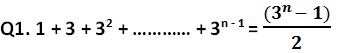Solution:

Let the given statement be P(n), i.e.,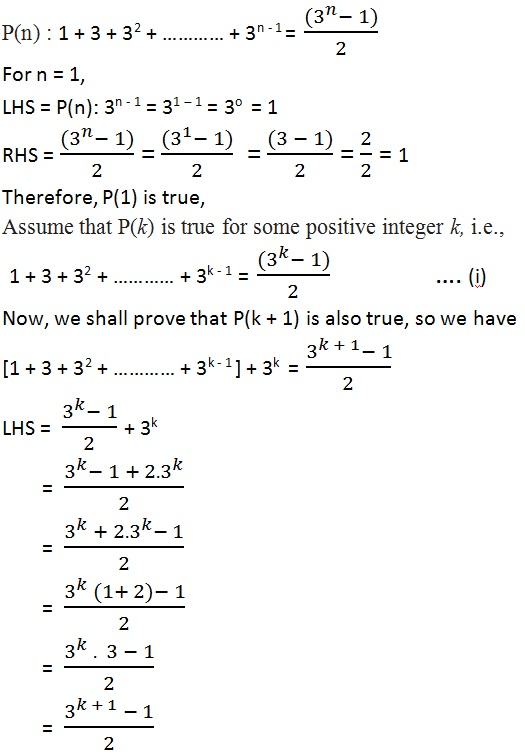LHS = RHS

Thus P(k + 1) is true, whenever P (k) is true.

Hence, from the principle of mathematical induction, the statement P(n) is true for all natural numbers n.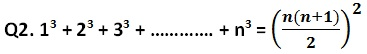Solution:

Let the given statement be P(n), i.e.,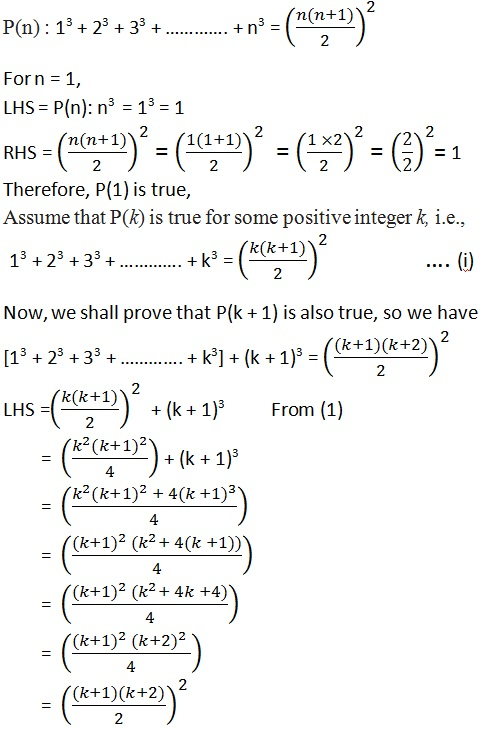LHS = RHS

Thus P(k + 1) is true, whenever P (k) is true.

Hence, from the principle of mathematical induction, the statement P(n) is true for all natural numbers n.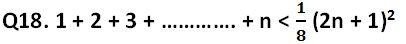Solution: Let the given statement be P(n), so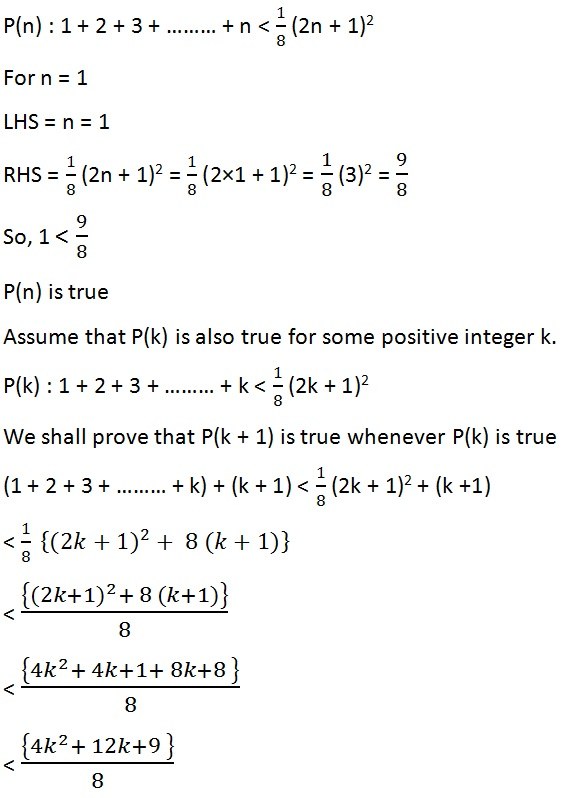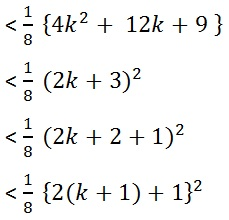Thus P(k + 1) is true, whenever P (k) is true.

Hence, from the principle of mathematical induction, the statement P(n) is true for all natural numbers n.

Q19. n (n + 1) (n + 5) is a multiple of 3.

Solution:

Let the given statement be P(n), so

P(n) : n (n + 1) (n + 5) is a multiple of 3.

For n = 1, so we have;

n (n + 1) (n + 5) = 1 × 2 × 6 = 12 = 3 × 4

P(n) is true for n = 1

Assume that P(k) is also true for some positive integer k.

k(k + 1) (k + 5)

= k3 + 6k2 + 5 k = 3m (say)   ……………….. (1)

Now, we shall prove that P(k + 1) is true whenever P(k) is true

Replacing k by k + 1

k + 1 (k + 2) (k + 6)

= (k + 1) (k2 + 8k + 12)

= k (k2 + 8k + 12) + 1(k2 + 8k + 12)

= k3 + 8k2 + 12k + k2 + 8k + 12

= k3 + 9k2 + 20k + 12

=( k3 + 6k2 + 5 k) + 3k2 + 15k + 12

= 3m + 3k2 + 15k + 12     from (1)

= 3(m + k2 + 5k + 4)

∴  k + 1 (k + 2) (k + 6) is multiple of 3

Thus P(k + 1) is true, whenever P (k) is true.

Hence, from the principle of mathematical induction, the statement P(n) is true for all natural numbers n ∈ N.

Q20.  102n - 1  + 1 is divisible by 11.

Solution:

Let the given statement be P(n), so

P(n) : 102n - 1  + 1 is divisible by 11.

For n = 1, so we have;

102n - 1  + 1 = 102×1 - 1 + 1 = 10 + 1 = 11

P(n) is true for n = 1

Assume that P(k) is also true for some positive integer k.

102k- 1  + 1  = 11m say

102k- 1  = 11m - 1      ……………… (1)

We shall prove that P(k + 1) is true whenever P(k) is true

replacing k by k + 1 we have

102k - 1  + 1

=  102k + 1  + 1

= 102k × 101 + 1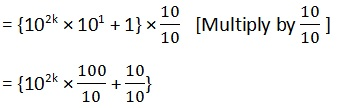= {102k - 1 × 100 + 1}

= {(11m - 1)× 100 + 1}     from equation (1)

= 1100m - 100+ 1

= 1100m - 99

= 11(100m - 9)

102n - 1  + 1 is divisible by 11

Thus P(k + 1) is true, whenever  P(k) is true.

Hence, from the principle of mathematical induction, the statement P(n) is true for all natural numbers n N.

Q21.  x2n y2n is divisible by x + y

Solution: Let the given statement be P(n), so

P(n) : x2n – y2n is divisible by x + y

Putting n = 1 we have,

x2n – y2n = x2 - y2 = (x + y) (x - y)

P(n) is true for n = 1

Assume that P(k) is also true for some positive integer k or

x2k – y2k is divisible by (x + y)

So, x2k – y2k = m( x + y)

Or  x2k = m( x + y) + y2k     …………. (1)

We shall prove that P(k + 1) is true whenever P(k) is true

replacing k by k + 1 we have

x2k + 2 – y2k + 2

= x2k . x2  – y2k .y2

Putting the value of x2k from (1)

= {m( x + y) + y2k} x2  – y2k .y2

= m( x + y) x2 + y2k. x2  – y2k .y2

= m( x + y) x2 + y2k (x2  – y2)

= m( x + y) x2 + y2k (x + y) ( x - y)

= ( x + y) [mx2 + y2k ( x - y)]

x2n – y2n is divisible by x + y

Thus P(k + 1) is true, whenever  P(k) is true.

Hence, from the principle of mathematical induction, the statement P(n) is true for all natural numbers n N.

Q22.  32n+2 – 8n – 9 is divisible by 8

Solution: Let the given statement be P(n), so

P(n) : 32n+2 – 8n – 9 is divisible by 8
Putting n =1

P(1) : 32×1+2 – 8 × 1 – 9 = 81 - 17 = 64 = 8 × 8

Which is divisible by 8

P(1) is true

Assume that P(k) is also true for some positive integer k

32k + 2 – 8k – 9

32k + 2 – 8k – 9 is divisible by 8
32k + 2 – 8k – 9 = 8m

Or 32k + 2 = 8m + 8k + 9     ……………. (1)

We shall prove that P(k + 1) is true whenever P(k) is true

replacing k by k + 1 we have

32k + 4 – 8k 8  – 9

= 32k + 4 – 8k 17

= 32k + 2 × 32 – 8k 17

= (8m + 8k + 9)× 9 – 8k 17

= 72m + 72k + 81 – 8k 17

= 72m + 64k + 64

= 8(9m + 8k + 8)

∴  32n+2 – 8n – 9 is divisible by 8

Thus P(k + 1) is true, whenever  P(k) is true.

Hence, from the principle of mathematical induction, the statement P(n) is true for all natural numbers n N.

Q23. 41n – 14n is a multiple of 27.

Solution: Let the given statement be P(n), so

P(n) : 41n – 14n is a multiple of 27
Putting n = 1

P(1): 41n – 14n = 41 – 14 = 27

P(1) is true

Assume that P(k) is also true for some positive integer k

41k – 14k = 27

41k = 27 + 14k   ………… (1)

We shall prove that P(k + 1) is true whenever P(k) is true

replacing k by k + 1 we have

41k + 1 – 14k + 1

=  41k . 41 – 14k . 14

=  (27 + 14k) 41 – 14k . 14

=  27 . 41 + 14k .41 – 14k . 14

=  27 . 41 + 14k (41 – 14 )

=  27 . 41 + 14k . 27

=  27 ( 41 + 14k )

41n – 14n is a multiple of 27
Thus P(k + 1) is true, whenever  P(k) is true.

Hence, from the principle of mathematical induction, the statement P(n) is true for all natural numbers n N.

Q24. (2n + 7) < (n + 3)2

Solution: Let the statement be p(n) so,

p(n) : (2n + 7) < (n + 3)2

=> p(1) :  (2 × 1 + 7) < (1 + 3)2

=> 9 < 42

=> 9 < 16

Therefore, p(1) is true so Assume that p(k) is also true for some integer k.

(2k + 7) < (k + 3)2  ......... (i)

Now we shall prove for p(k + 1)

2(k +1) + 7 < (k + 1 + 3)2

2k + 2 + 7 < (k + 4)2  ........ (ii)

We have from (i)

(2k + 7) < (k + 3)2

=> 2k + 7 + 2 < (k + 3)2 + 2

=> 2k + 7 + 2 < k2 + 6k + 9 + 2

=> 2k + 7 + 2 < k2​ + 6k + 9 + 2

=> 2k + 7 + 2 < k2​ + 6k + 11

Now, k2​ + 6k + 11 < (k + 4)2 from (ii)

=> 2k + 7 + 2 < k+ 6k + 11 < k2 + 8k + 16

=> 2k + 2 + 7 < k2 + 8k + 16

=> 2(k + 1) + 7 < (k + 4)2

=> 2(k + 1) + 7 < (k + 1 + 3)2

Thus P(+ 1) is true, whenever  P(k) is true.

Hence, from the principle of mathematical induction, the statement P(n) is true for all natural numbers n ∈ N.

Chapter Contents: